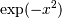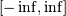# numpy.polynomial.hermite.hermweight¶

numpy.polynomial.hermite.hermweight(x)[source]

Weight function of the Hermite polynomials.

The weight function isand the interval of integration is. the Hermite polynomials are orthogonal, but not normalized, with respect to this weight function.

Parameters: x : array_like Values at which the weight function will be computed. w : ndarray The weight function at x.

Notes

#### Previous topic

numpy.polynomial.hermite.hermgauss

#### Next topic

numpy.polynomial.hermite.hermcompanion